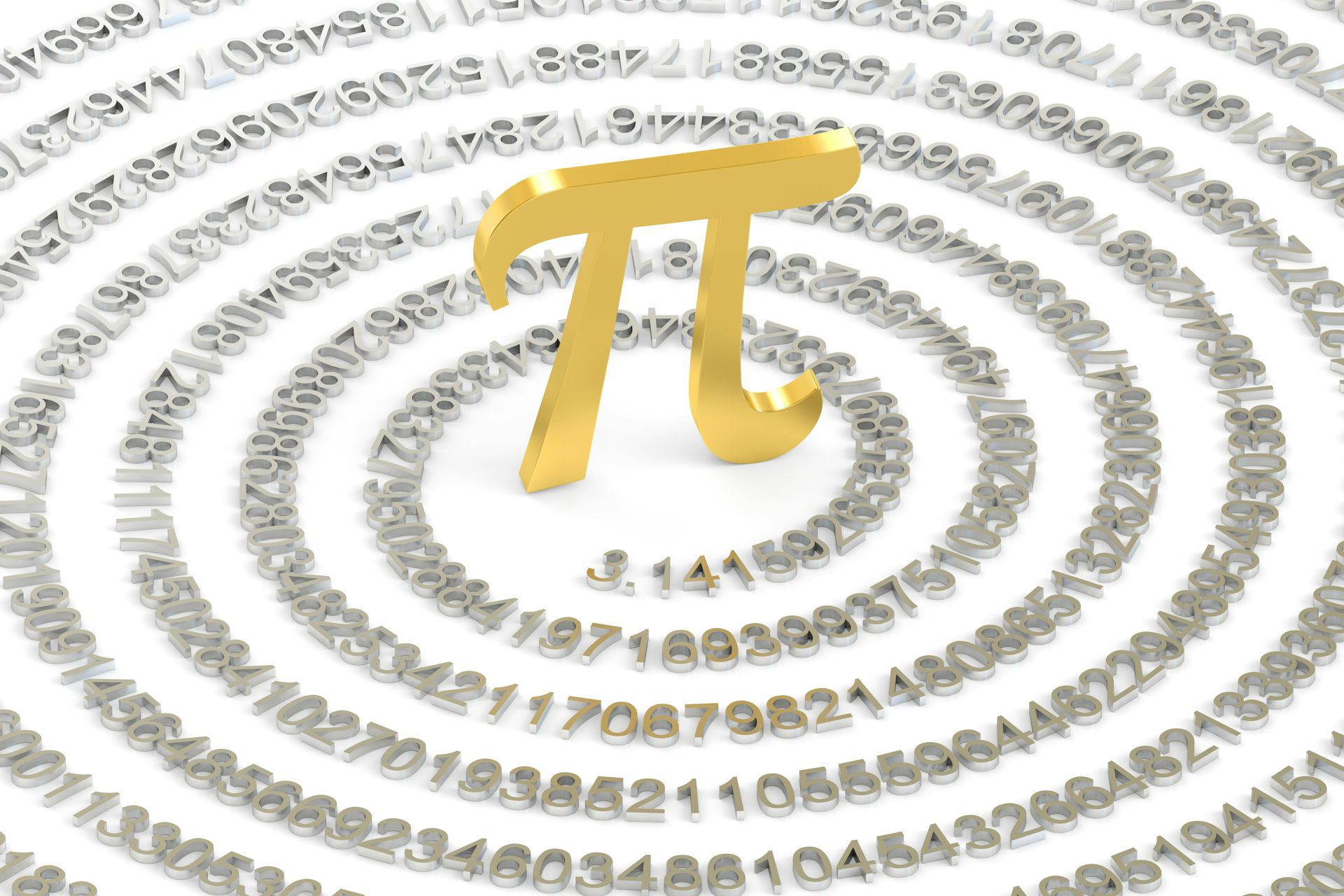Shutterstock

After thousands of years of trying, mathematicians are still working out the number known as pi or “π”. We typically think of pi as approximately 3.14 but the most successful attempt to calculate it more precisely worked out its value to over 13 trillion digits after the decimal point. We have known since the 18th century that we will never be able to calculate all the digits of pi because it is an irrational number, one that continues forever without any repeating pattern.

In 1888, the logician John Venn, who also invented the Venn diagram, attempted to visually show that the digits of pi were random by drawing a graph showing the first 707 decimal places. He assigned a compass point to the digits 0 to 7 and then drew lines to show the path indicated by each digit.

Venn did this work using pen and paper but this is still used today with modern technology to create even more detailed and beautiful patterns.

But, despite the endless string of unpredictable digits that make up pi, it’s not what we call a truly random number. And it actually contains all sorts of surprising patterns.

## Normal not random

The reason we can’t call pi random is because the digits it comprises are precisely determined and fixed. For example, the second decimal place in pi is always 4. So you can’t ask what the probability would be of a different number taking this position. It isn’t randomly positioned.

But we can ask the related question: “Is pi a normal number?” A decimal number is said to be normal when every sequence of possible digits is equally likely to appear in it, making the numbers look random even if they technically aren’t. By looking at the digits of pi and applying statistical tests you can try to determine if it is normal. From the tests performed so far, it is still an open question whether pi is normal or not.

For example in 2003, Yasumasa Kanada published the distribution of the number of times different digits appear in the first trillion digits of pi:

``````    Digit       Occurrences
0           99,999,485,134
1           99,999,945,664
2           100,000,480,057
3           99,999,787,805
4           100,000,357,857
5           99,999,671,008
6           99,999,807,503
7           99,999,818,723
8           100,000,791,469
9           99,999,854,780
Total       1,000,000,000,000
``````

His results imply that these digits seem to be fairly evenly distributed, but it is not enough to prove that all of pi would be normal.

## Every sequence

We need to remember the surprising fact that if pi was normal then any finite sequence of digits you could name could be found in it. For example, at position 768 in the pi digits there are six 9s in succession. The chance of this happening if pi is normal and every sequence of n digits is equally likely to occur, is 0.08%.

This block of nines is famously called the “Feynman Point” after the Nobel Prize-winner Richard Feynman. He once jokingly claimed that if he had to recite pi digits he would name them up to this point and then say “and so on”.

Other interesting sequences of digits have also been found. At position 17,387,594,880 you find the sequence 0123456789, and surprisingly earlier at position 60 you find these ten digits in a scrambled order.

Pi-hunters search for dates of birth and other significant personal numbers in pi asking the question: “Where do I occur in the pi digits?” If you want to test to see where your own special numbers are in pi, then you can do so by using the free online software called Pi birthdays.

### Want to write?

Write an article and join a growing community of more than 88,500 academics and researchers from 2,928 institutions.